Скачать презентацию Chapter 18 Multiple Regression 18 1 Introduction

acf2104bb6c853d6f44a412b5f5547f2.ppt

• Количество слайдов: 45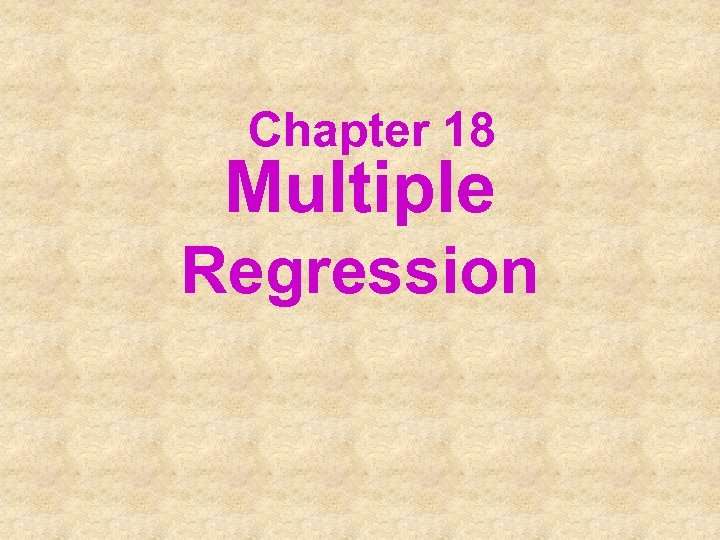Chapter 18 Multiple Regression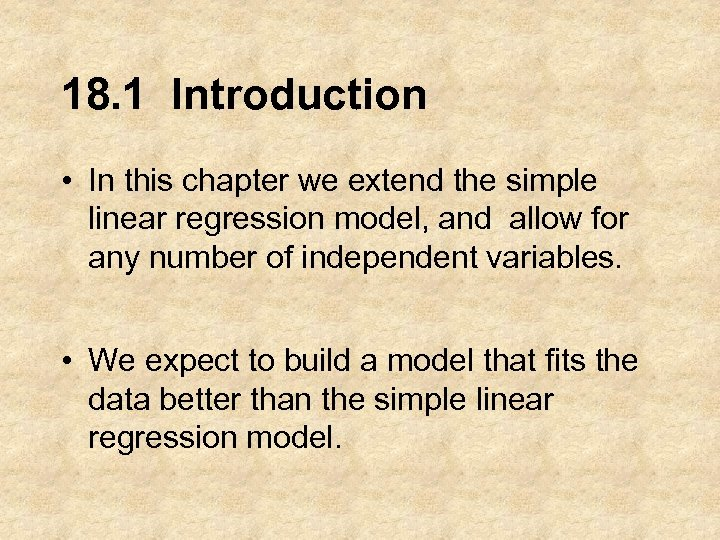18. 1 Introduction • In this chapter we extend the simple linear regression model, and allow for any number of independent variables. • We expect to build a model that fits the data better than the simple linear regression model.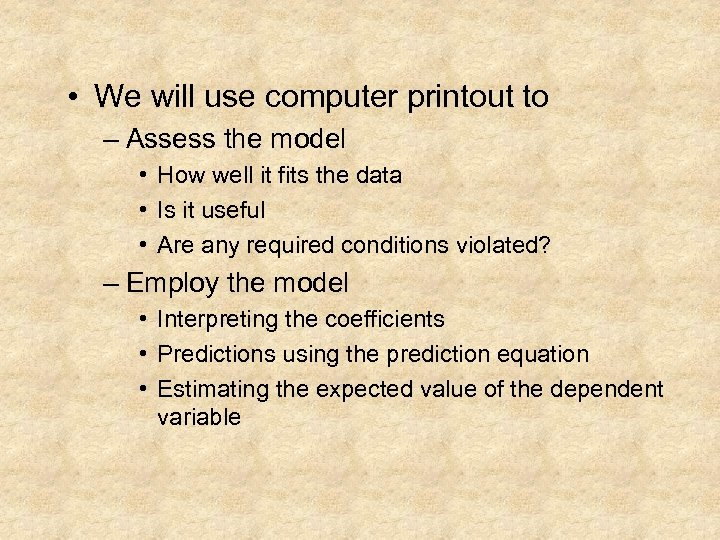• We will use computer printout to – Assess the model • How well it fits the data • Is it useful • Are any required conditions violated? – Employ the model • Interpreting the coefficients • Predictions using the prediction equation • Estimating the expected value of the dependent variable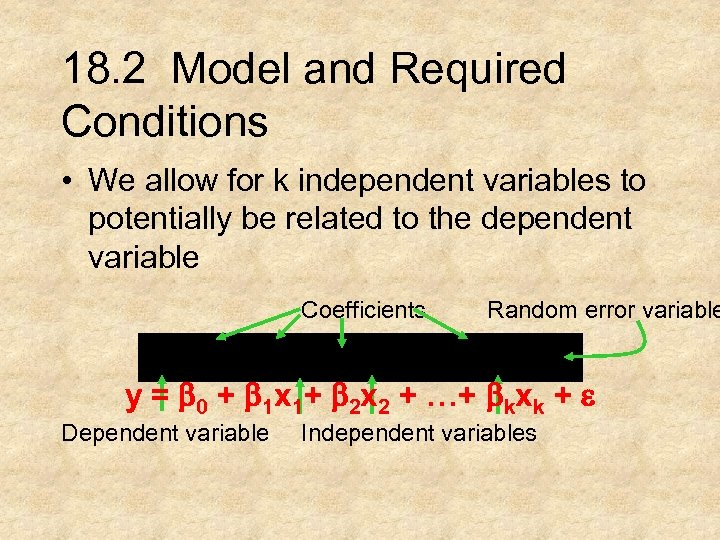18. 2 Model and Required Conditions • We allow for k independent variables to potentially be related to the dependent variable Coefficients Random error variable y = b 0 + b 1 x 1+ b 2 x 2 + …+ bkxk + e Dependent variable Independent variables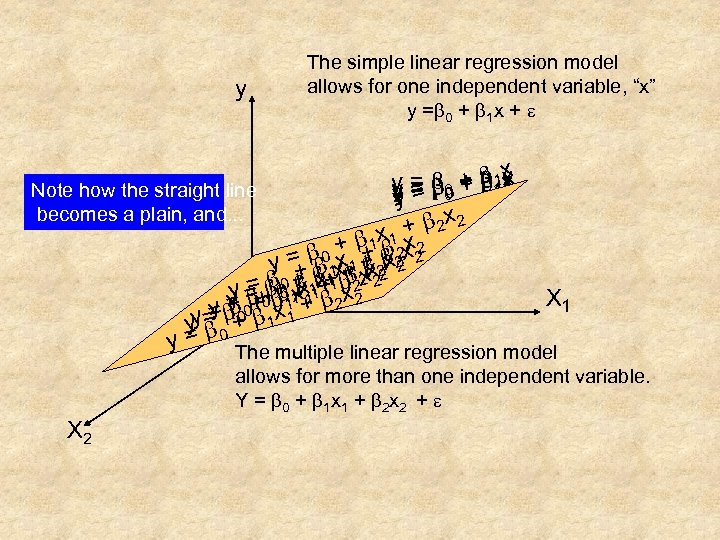y The simple linear regression model allows for one independent variable, “x” y =b 0 + b 1 x + e b 1 x y = b 0 + b 1 x Note how the straight line y = b 0 y= 0 becomes a plain, and. . . + b 2 x 2 + b 1 x 12 x 2 b b 0 x 1 + b 2 x 2 y = b 1 x+ + 2 x 2 b + x 1 b x b+ + 1 b+b 122 x 22 0 x = bb x y =+b 0 1 b 11+1 x 2 X 1 y + = b b 1 y b 00 b 0 x 1 + b 2 y= y =0 + 1 y = b The multiple linear regression model allows for more than one independent variable. Y = b 0 + b 1 x 1 + b 2 x 2 + e X 2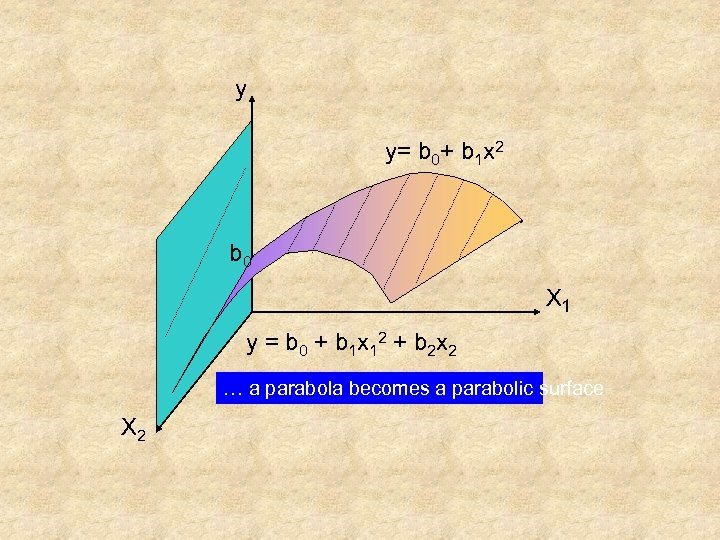y y= b 0+ b 1 x 2 b 0 X 1 y = b 0 + b 1 x 12 + b 2 x 2 … a parabola becomes a parabolic surface X 2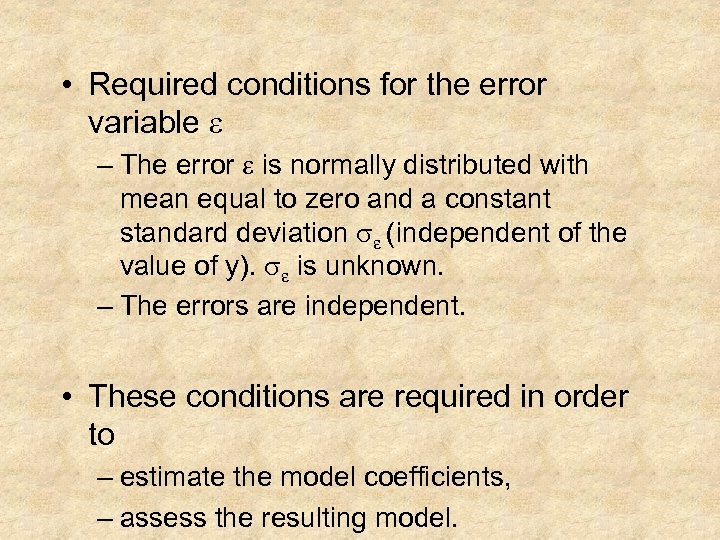• Required conditions for the error variable e – The error e is normally distributed with mean equal to zero and a constant standard deviation se (independent of the value of y). se is unknown. – The errors are independent. • These conditions are required in order to – estimate the model coefficients, – assess the resulting model.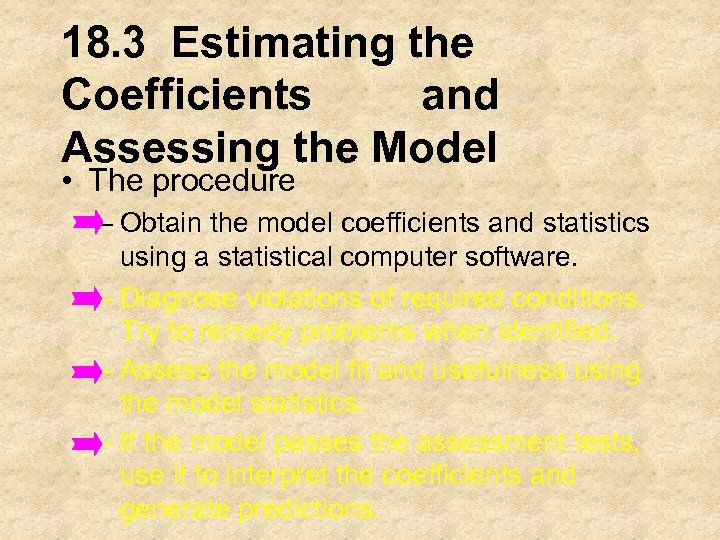18. 3 Estimating the Coefficients and Assessing the Model • The procedure – Obtain the model coefficients and statistics using a statistical computer software. – Diagnose violations of required conditions. Try to remedy problems when identified. – Assess the model fit and usefulness using the model statistics. – If the model passes the assessment tests, use it to interpret the coefficients and generate predictions.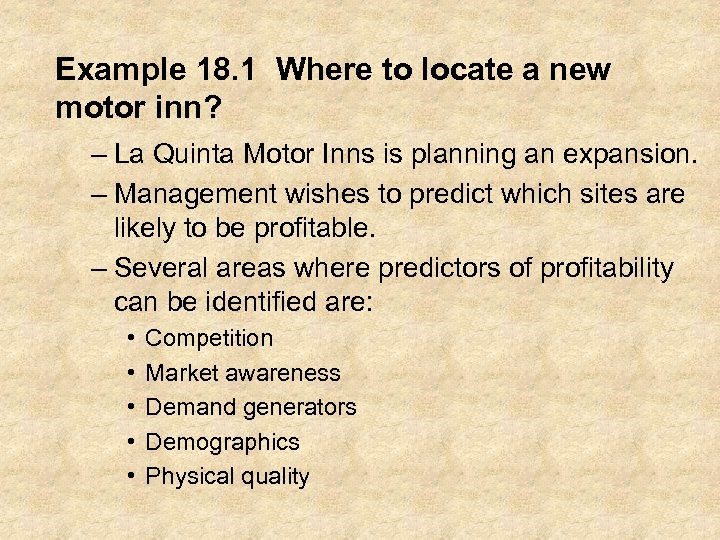Example 18. 1 Where to locate a new motor inn? – La Quinta Motor Inns is planning an expansion. – Management wishes to predict which sites are likely to be profitable. – Several areas where predictors of profitability can be identified are: • • • Competition Market awareness Demand generators Demographics Physical quality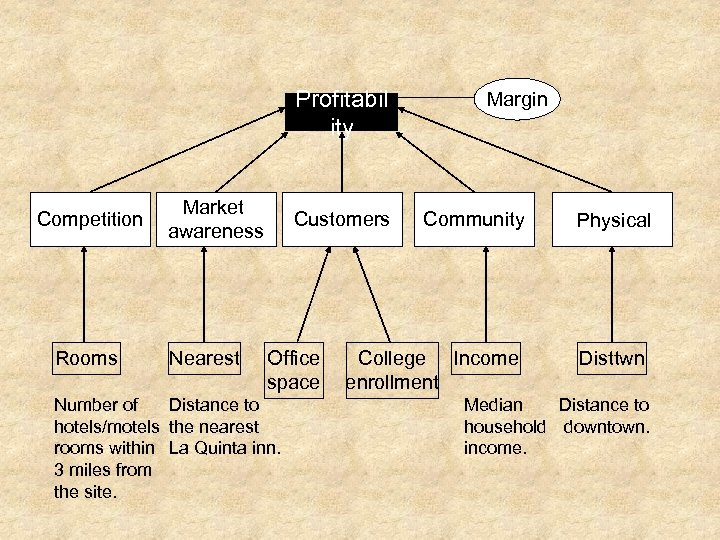Profitabil ity Competition Rooms Market awareness Nearest Customers Office space Distance to Number of hotels/motels the nearest rooms within La Quinta inn. 3 miles from the site. Margin Community College Income enrollment Physical Disttwn Median Distance to household downtown. income.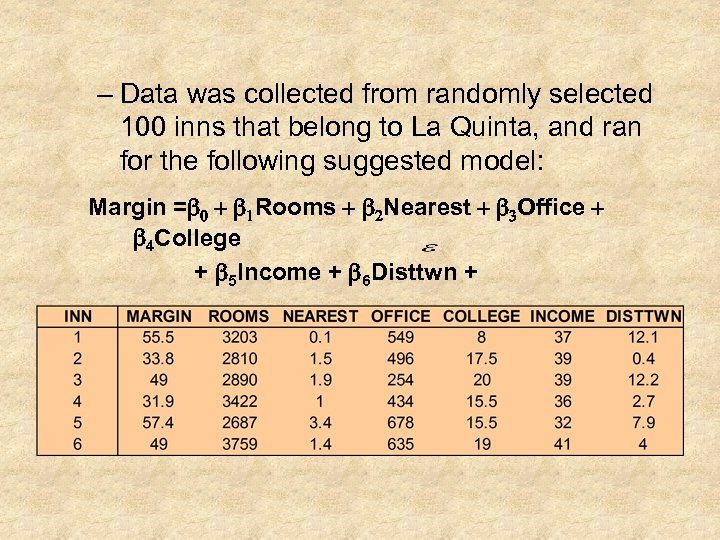– Data was collected from randomly selected 100 inns that belong to La Quinta, and ran for the following suggested model: Margin =b 0 + b 1 Rooms + b 2 Nearest + b 3 Office + b 4 College + b 5 Income + b 6 Disttwn +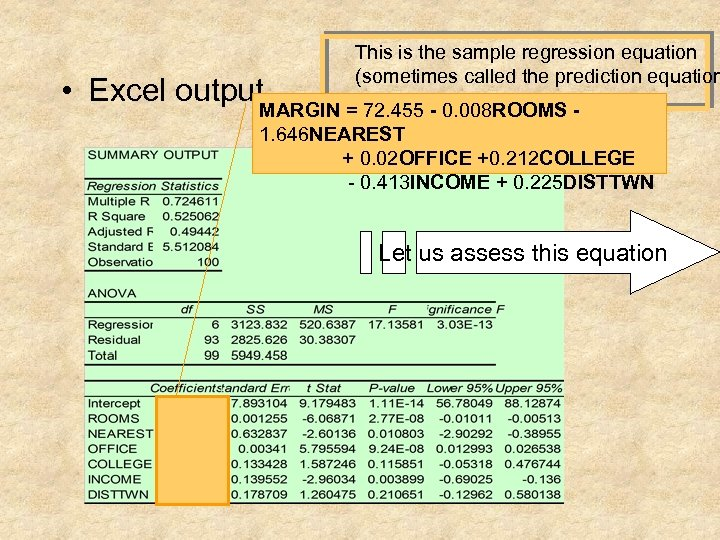This is the sample regression equation (sometimes called the prediction equation • Excel output. MARGIN = 72. 455 - 0. 008 ROOMS - 1. 646 NEAREST + 0. 02 OFFICE +0. 212 COLLEGE - 0. 413 INCOME + 0. 225 DISTTWN Let us assess this equation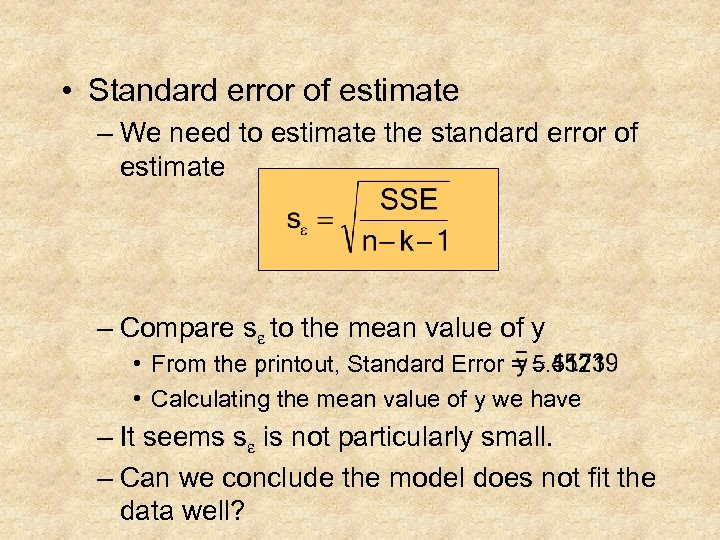• Standard error of estimate – We need to estimate the standard error of estimate – Compare se to the mean value of y • From the printout, Standard Error = 5. 5121 • Calculating the mean value of y we have – It seems se is not particularly small. – Can we conclude the model does not fit the data well?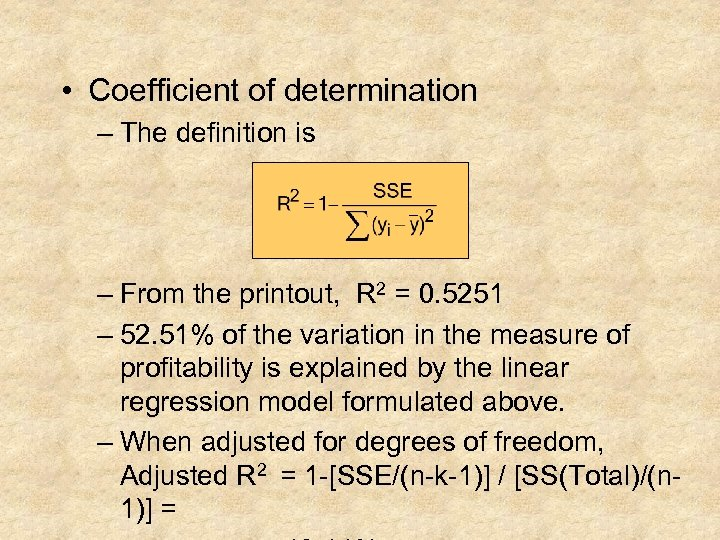• Coefficient of determination – The definition is – From the printout, R 2 = 0. 5251 – 52. 51% of the variation in the measure of profitability is explained by the linear regression model formulated above. – When adjusted for degrees of freedom, Adjusted R 2 = 1 -[SSE/(n-k-1)] / [SS(Total)/(n 1)] =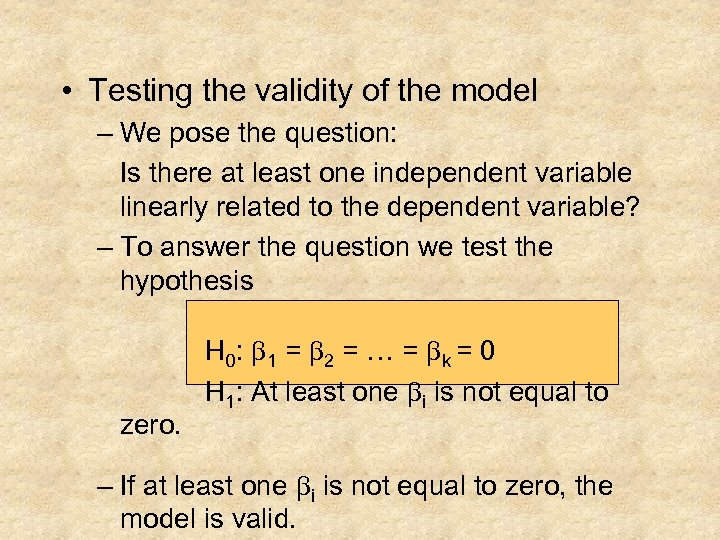• Testing the validity of the model – We pose the question: Is there at least one independent variable linearly related to the dependent variable? – To answer the question we test the hypothesis zero. H 0: b 1 = b 2 = … = b k = 0 H 1: At least one bi is not equal to – If at least one bi is not equal to zero, the model is valid.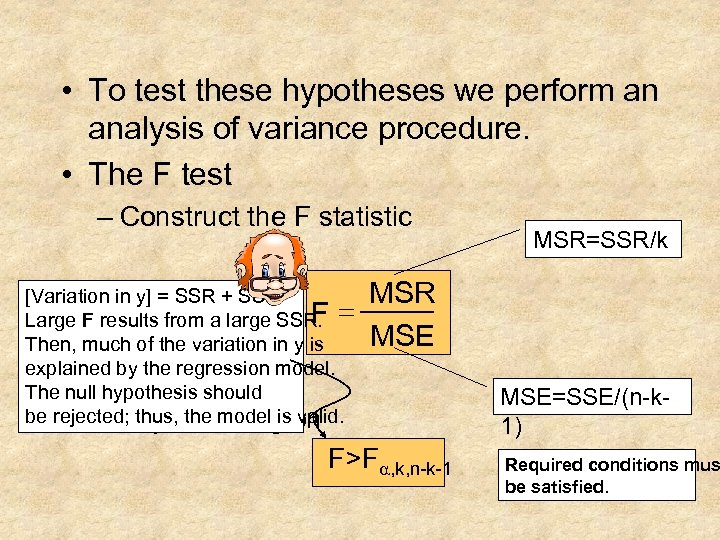• To test these hypotheses we perform an analysis of variance procedure. • The F test – Construct the F statistic [Variation in y] = SSR + SSE. F Large F results from a large SSR. = Then, much of the variation in y is explained by the regression model. The null hypothesis should be rejected; thus, the model is valid. – Rejection region MSR=SSR/k MSR MSE F>Fa, k, n-k-1 MSE=SSE/(n-k 1) Required conditions mus be satisfied.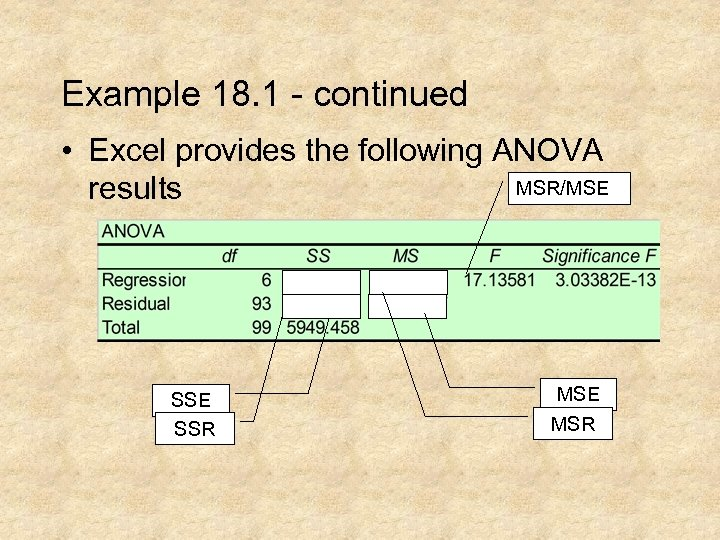Example 18. 1 - continued • Excel provides the following ANOVA MSR/MSE results SSE SSR MSE MSR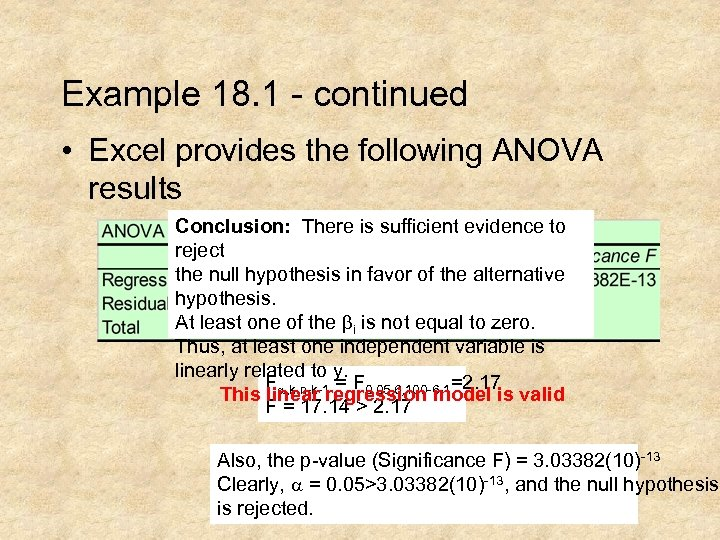Example 18. 1 - continued • Excel provides the following ANOVA results Conclusion: There is sufficient evidence to reject the null hypothesis in favor of the alternative hypothesis. At least one of the bi is not equal to zero. Thus, at least one independent variable is linearly related to y. Fa, k, n-k-1 = F 0. 05, 6, 100 -6 -1=2. 17 This linear regression model is valid F = 17. 14 > 2. 17 Also, the p-value (Significance F) = 3. 03382(10)-13 Clearly, a = 0. 05>3. 03382(10)-13, and the null hypothesis is rejected.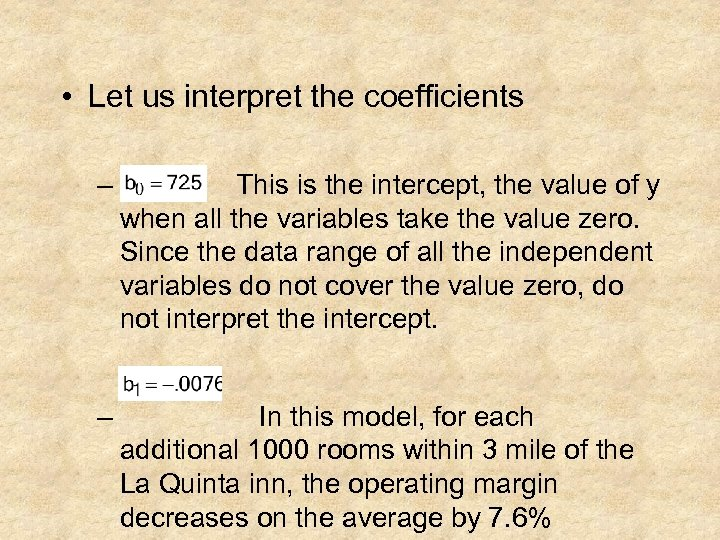• Let us interpret the coefficients – This is the intercept, the value of y when all the variables take the value zero. Since the data range of all the independent variables do not cover the value zero, do not interpret the intercept. – In this model, for each additional 1000 rooms within 3 mile of the La Quinta inn, the operating margin decreases on the average by 7. 6%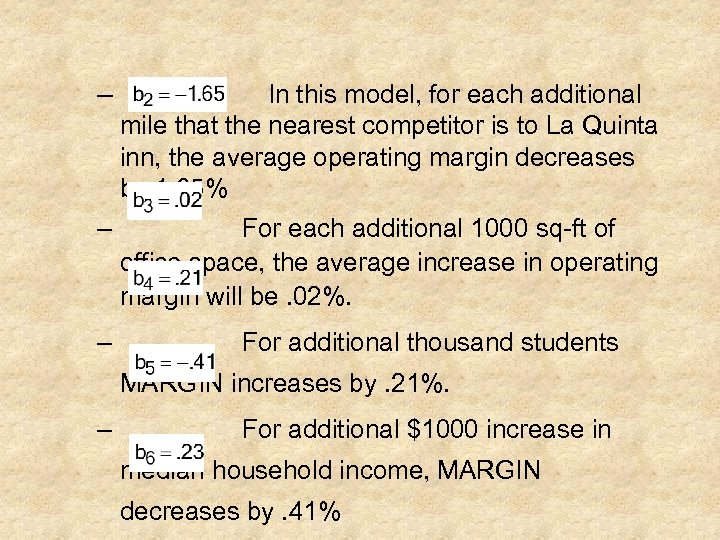– In this model, for each additional mile that the nearest competitor is to La Quinta inn, the average operating margin decreases by 1. 65% – For each additional 1000 sq-ft of office space, the average increase in operating margin will be. 02%. – For additional thousand students MARGIN increases by. 21%. – For additional \$1000 increase in median household income, MARGIN decreases by. 41%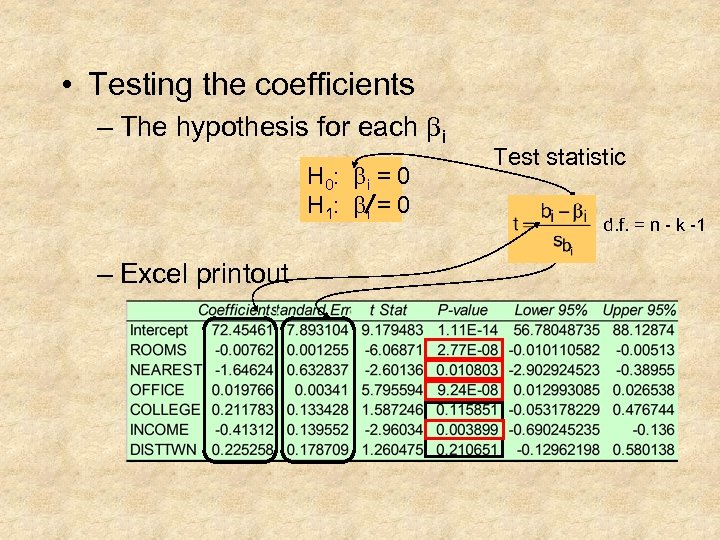• Testing the coefficients – The hypothesis for each bi H 0: b i = 0 H 1: b i = 0 – Excel printout Test statistic d. f. = n - k -1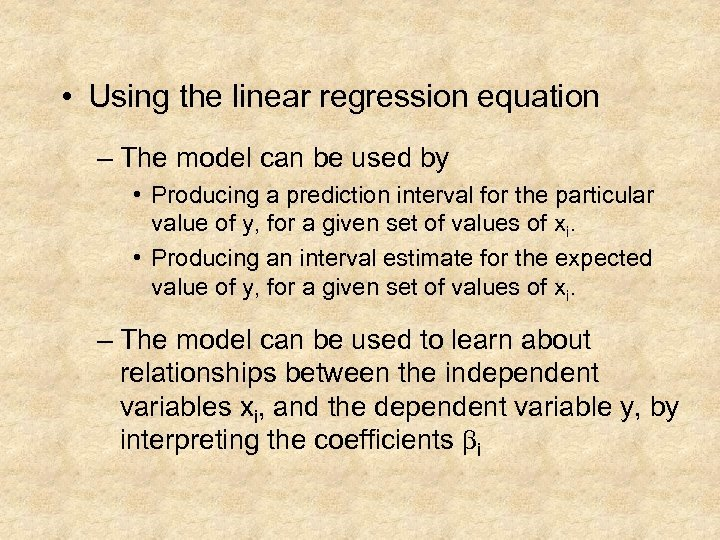• Using the linear regression equation – The model can be used by • Producing a prediction interval for the particular value of y, for a given set of values of xi. • Producing an interval estimate for the expected value of y, for a given set of values of xi. – The model can be used to learn about relationships between the independent variables xi, and the dependent variable y, by interpreting the coefficients bi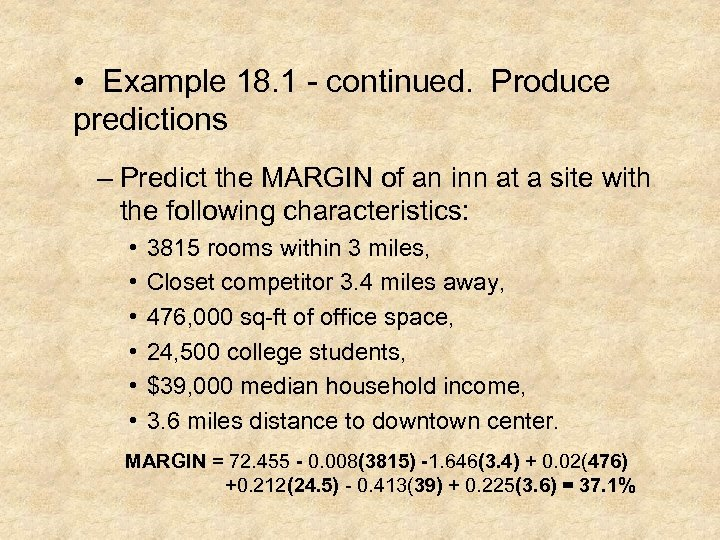• Example 18. 1 - continued. Produce predictions – Predict the MARGIN of an inn at a site with the following characteristics: • • • 3815 rooms within 3 miles, Closet competitor 3. 4 miles away, 476, 000 sq-ft of office space, 24, 500 college students, \$39, 000 median household income, 3. 6 miles distance to downtown center. MARGIN = 72. 455 - 0. 008(3815) -1. 646(3. 4) + 0. 02(476) +0. 212(24. 5) - 0. 413(39) + 0. 225(3. 6) = 37. 1%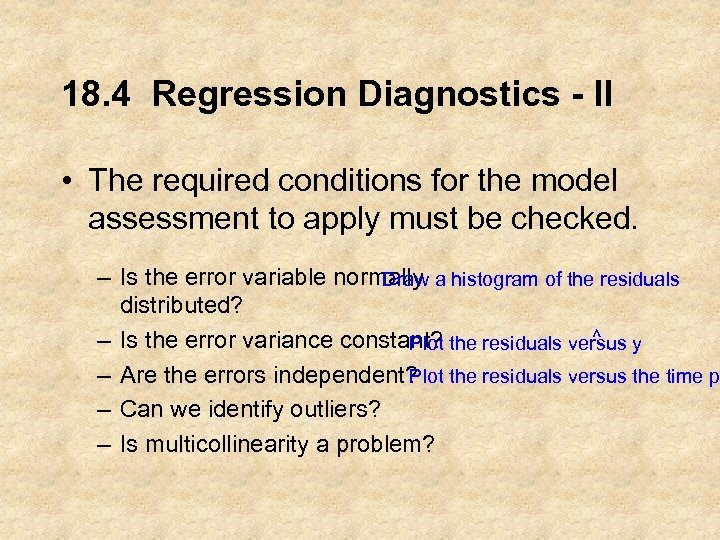18. 4 Regression Diagnostics - II • The required conditions for the model assessment to apply must be checked. – Is the error variable normally a histogram of the residuals Draw distributed? ^ – Is the error variance constant? the residuals versus y Plot – Are the errors independent? the residuals versus the time p Plot – Can we identify outliers? – Is multicollinearity a problem?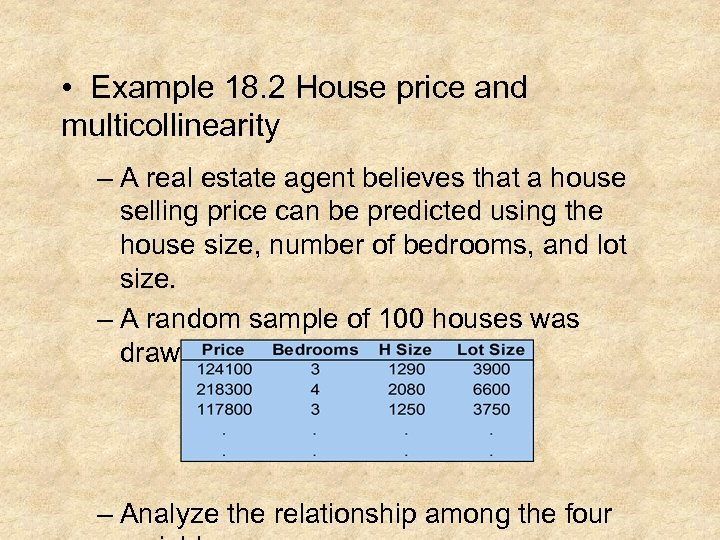• Example 18. 2 House price and multicollinearity – A real estate agent believes that a house selling price can be predicted using the house size, number of bedrooms, and lot size. – A random sample of 100 houses was drawn and data recorded. – Analyze the relationship among the four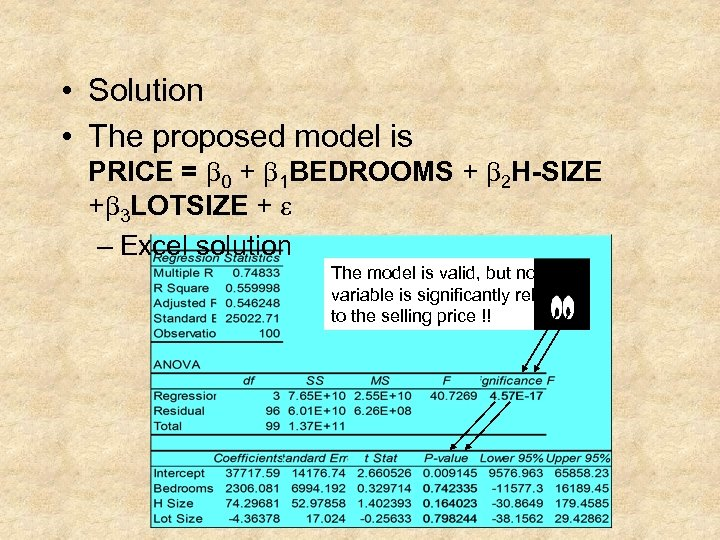• Solution • The proposed model is PRICE = b 0 + b 1 BEDROOMS + b 2 H-SIZE +b 3 LOTSIZE + e – Excel solution The model is valid, but no variable is significantly related to the selling price !!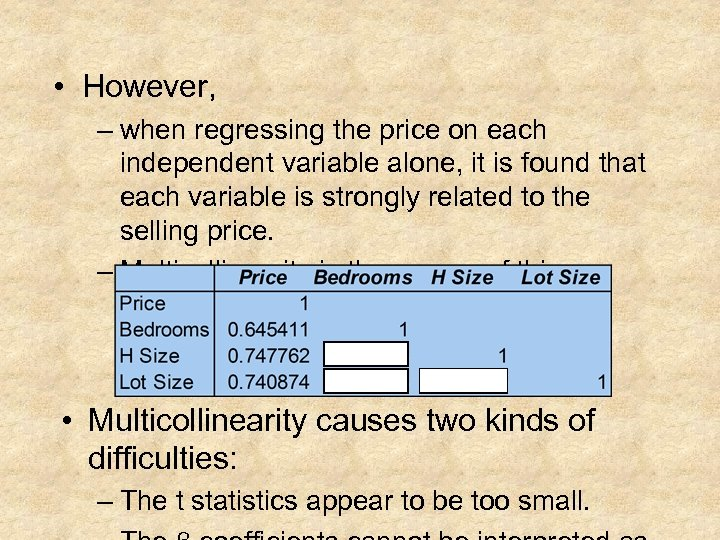• However, – when regressing the price on each independent variable alone, it is found that each variable is strongly related to the selling price. – Multicollinearity is the source of this problem. • Multicollinearity causes two kinds of difficulties: – The t statistics appear to be too small.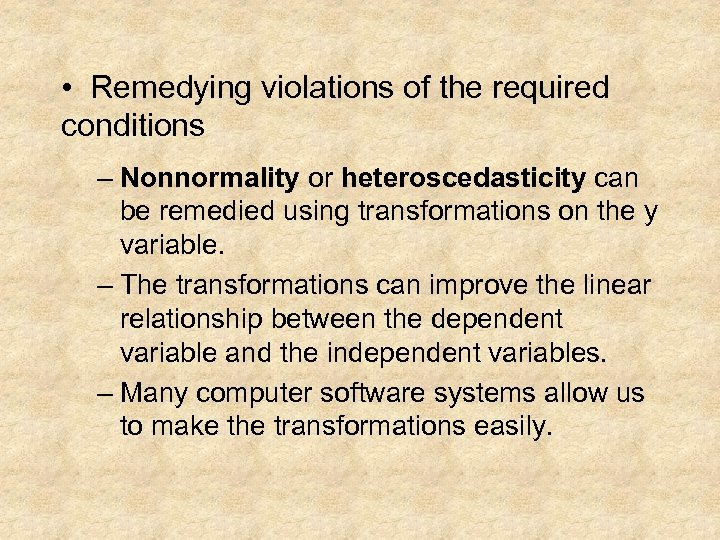• Remedying violations of the required conditions – Nonnormality or heteroscedasticity can be remedied using transformations on the y variable. – The transformations can improve the linear relationship between the dependent variable and the independent variables. – Many computer software systems allow us to make the transformations easily.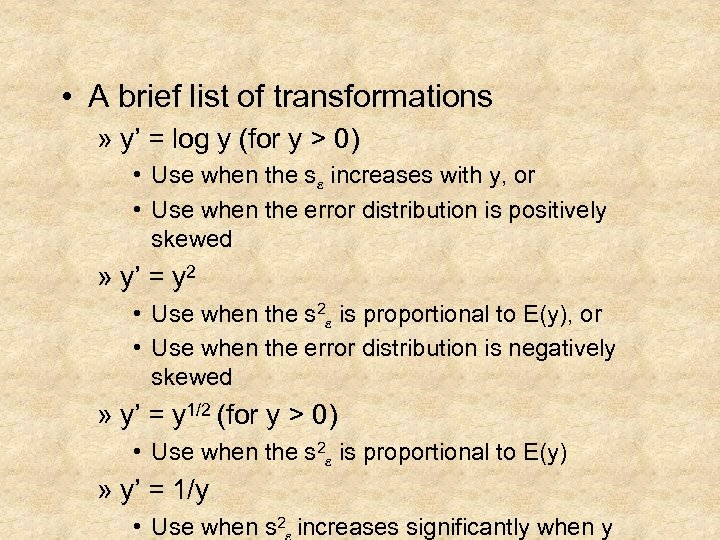• A brief list of transformations » y’ = log y (for y > 0) • Use when the se increases with y, or • Use when the error distribution is positively skewed » y’ = y 2 • Use when the s 2 e is proportional to E(y), or • Use when the error distribution is negatively skewed » y’ = y 1/2 (for y > 0) • Use when the s 2 e is proportional to E(y) » y’ = 1/y • Use when s 2 increases significantly when y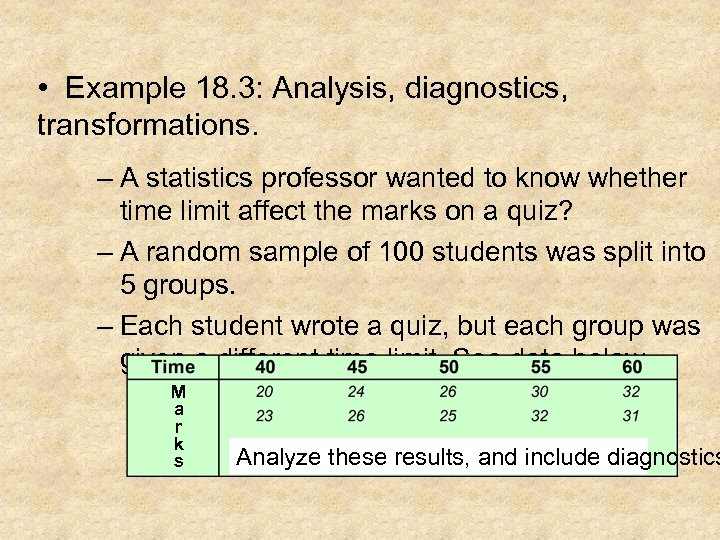• Example 18. 3: Analysis, diagnostics, transformations. – A statistics professor wanted to know whether time limit affect the marks on a quiz? – A random sample of 100 students was split into 5 groups. – Each student wrote a quiz, but each group was given a different time limit. See data below. M a r k s Analyze these results, and include diagnostics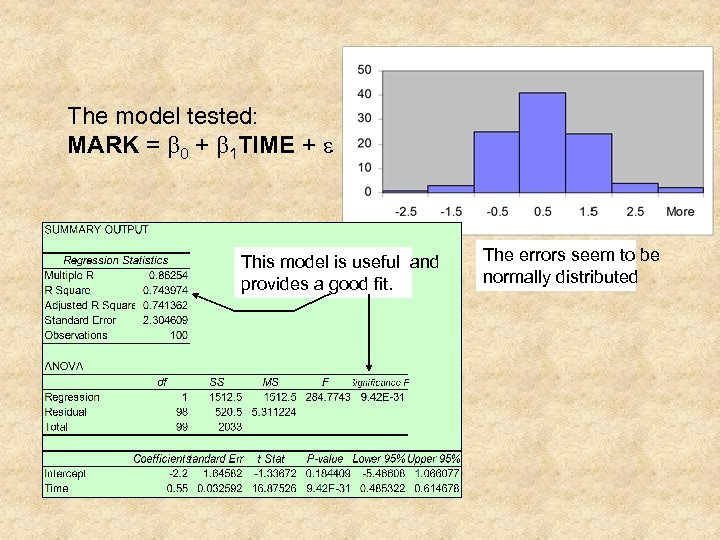The model tested: MARK = b 0 + b 1 TIME + e This model is useful and provides a good fit. The errors seem to be normally distributed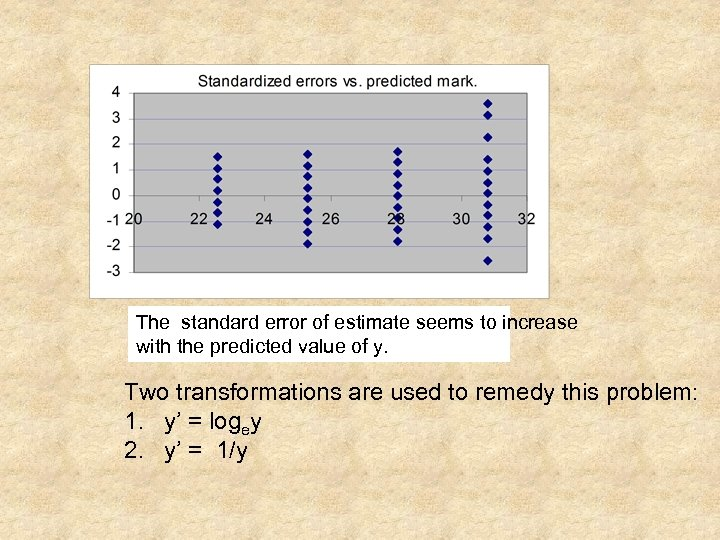The standard error of estimate seems to increase with the predicted value of y. Two transformations are used to remedy this problem: 1. y’ = logey 2. y’ = 1/y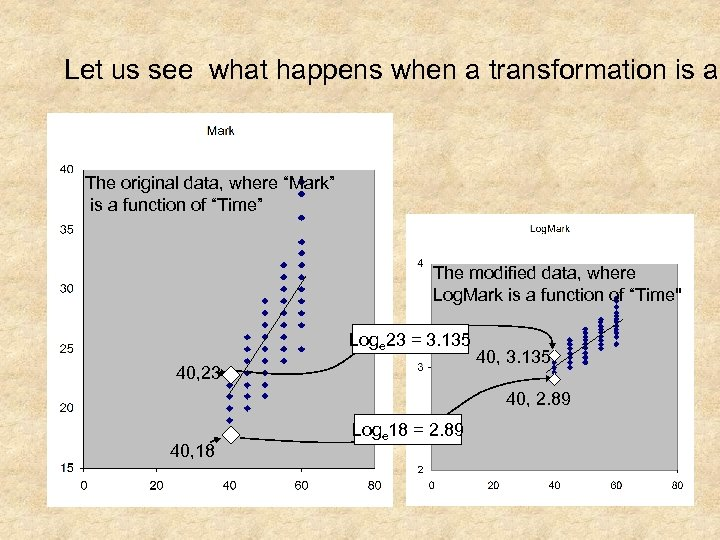Let us see what happens when a transformation is ap The original data, where “Mark” is a function of “Time” The modified data, where Log. Mark is a function of “Time" Loge 23 = 3. 135 40, 23 40, 3. 135 40, 2. 89 40, 18 Loge 18 = 2. 89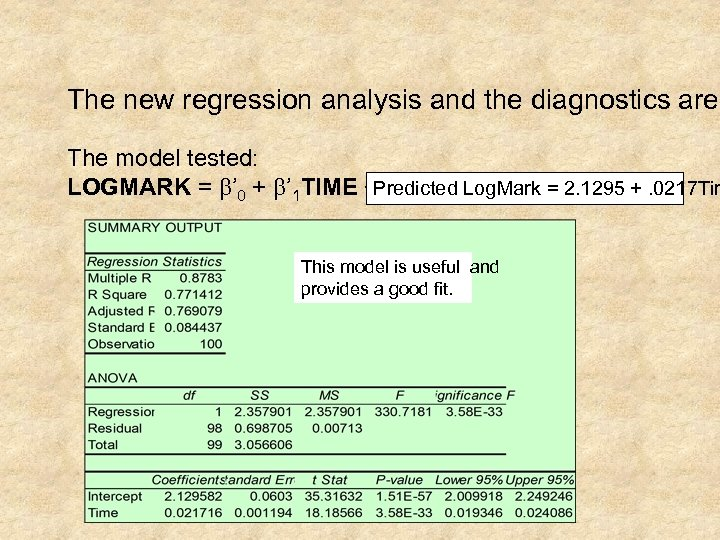The new regression analysis and the diagnostics are: The model tested: LOGMARK = b’ 0 + b’ 1 TIME + e’ Predicted Log. Mark = 2. 1295 +. 0217 Tim This model is useful and provides a good fit.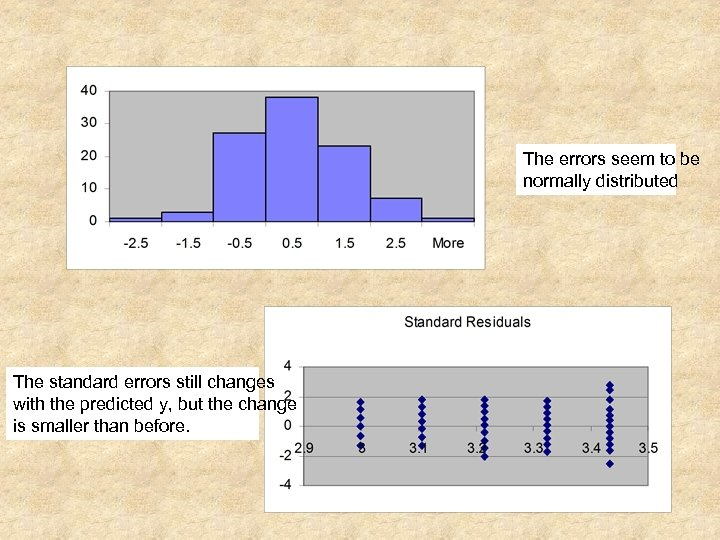The errors seem to be normally distributed The standard errors still changes with the predicted y, but the change is smaller than before.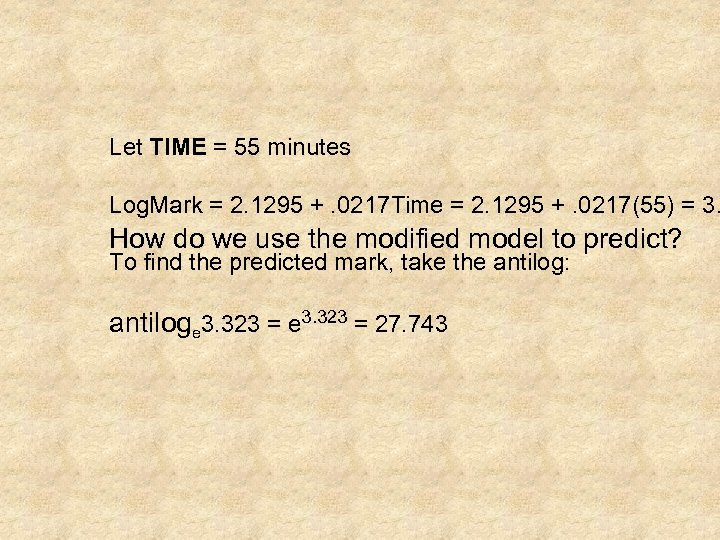Let TIME = 55 minutes Log. Mark = 2. 1295 +. 0217 Time = 2. 1295 +. 0217(55) = 3. How do we use the modified model to predict? To find the predicted mark, take the antilog: antiloge 3. 323 = 27. 743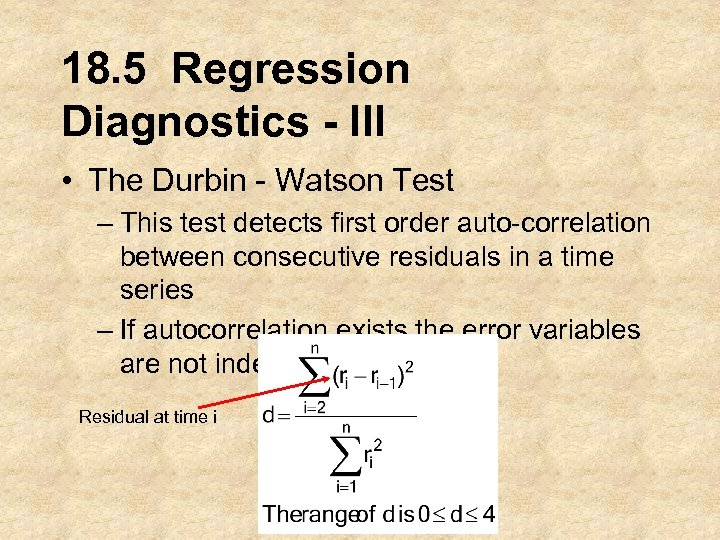18. 5 Regression Diagnostics - III • The Durbin - Watson Test – This test detects first order auto-correlation between consecutive residuals in a time series – If autocorrelation exists the error variables are not independent Residual at time i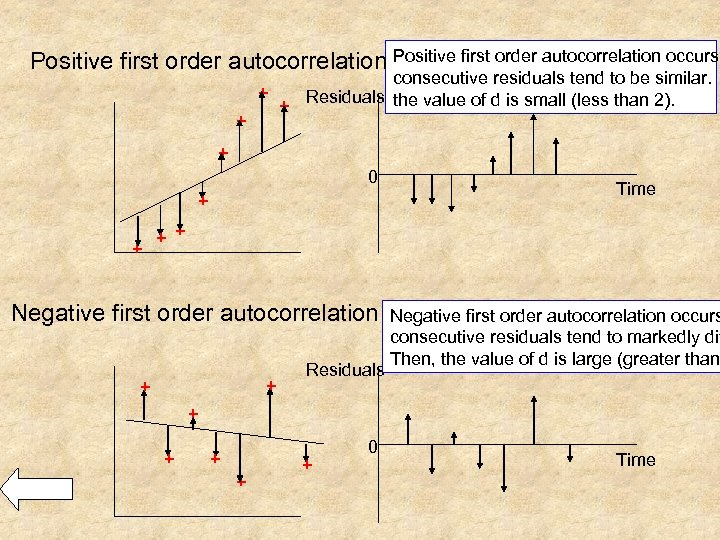Positive first order autocorrelation occurs + + consecutive residuals tend to be similar. + Residuals the value of d is small (less than 2). + 0 + + Time + + Negative first order autocorrelation + + Residuals Negative first order autocorrelation occurs consecutive residuals tend to markedly dif Then, the value of d is large (greater than + + + 0 Time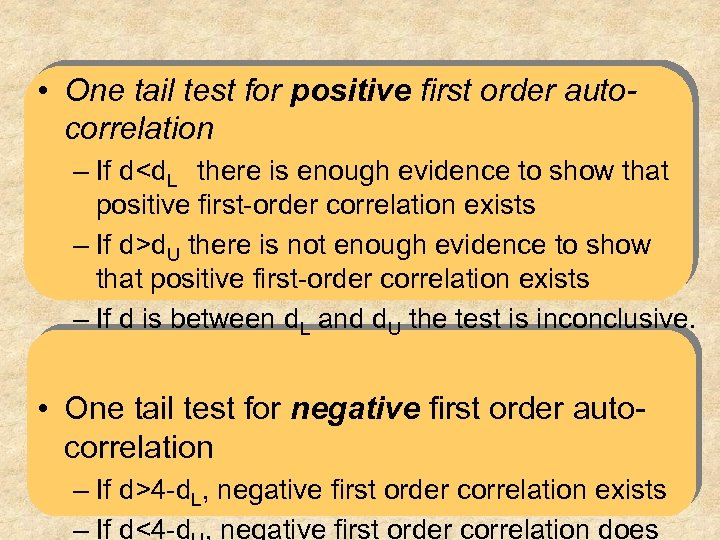• One tail test for positive first order autocorrelation – If dd. U there is not enough evidence to show that positive first-order correlation exists – If d is between d. L and d. U the test is inconclusive. • One tail test for negative first order autocorrelation – If d>4 -d. L, negative first order correlation exists – If d<4 -d , negative first order correlation does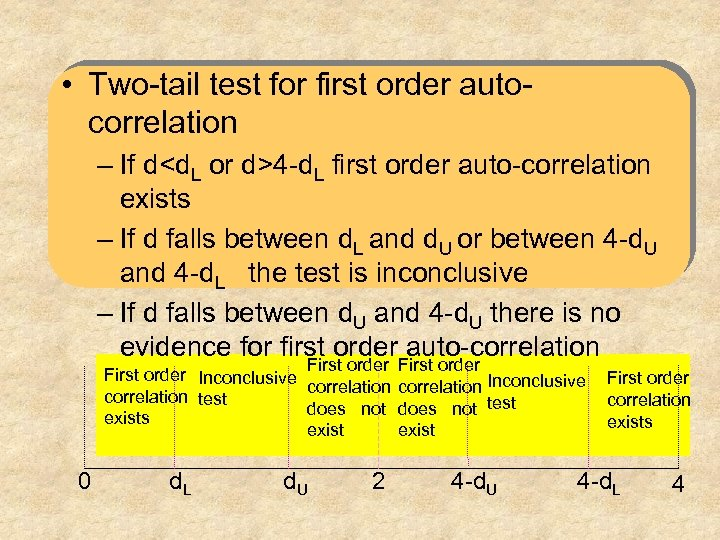• Two-tail test for first order autocorrelation – If d4 -d. L first order auto-correlation exists – If d falls between d. L and d. U or between 4 -d. U and 4 -d. L the test is inconclusive – If d falls between d. U and 4 -d. U there is no evidence for first order auto-correlation First order Inconclusive correlation test does not test exists exist 0 d. L d. U 2 4 -d. U First order correlation exists 4 -d. L 4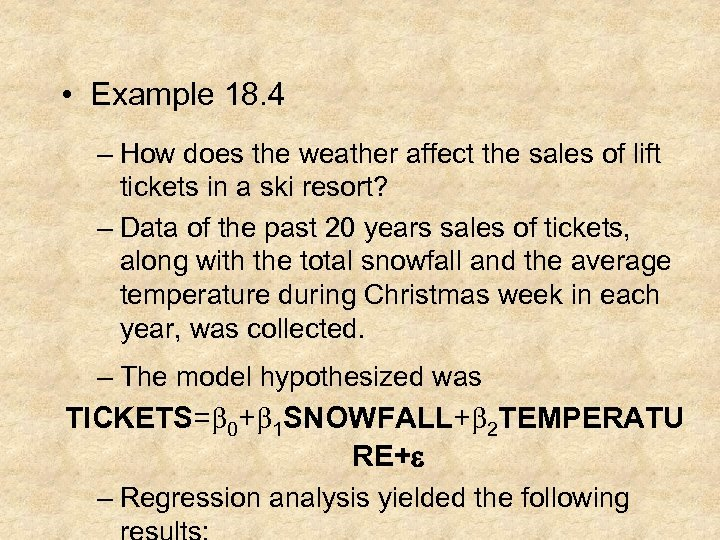• Example 18. 4 – How does the weather affect the sales of lift tickets in a ski resort? – Data of the past 20 years sales of tickets, along with the total snowfall and the average temperature during Christmas week in each year, was collected. – The model hypothesized was TICKETS=b 0+b 1 SNOWFALL+b 2 TEMPERATU RE+e – Regression analysis yielded the following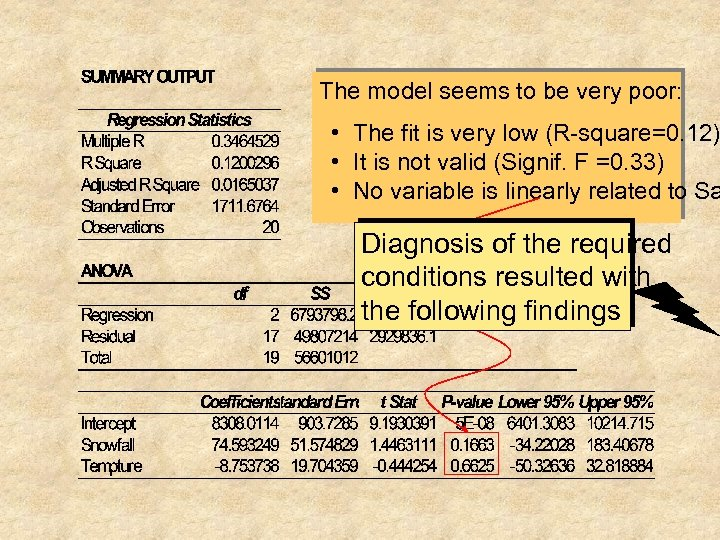The model seems to be very poor: • The fit is very low (R-square=0. 12) • It is not valid (Signif. F =0. 33) • No variable is linearly related to Sa Diagnosis of the required conditions resulted with the following findings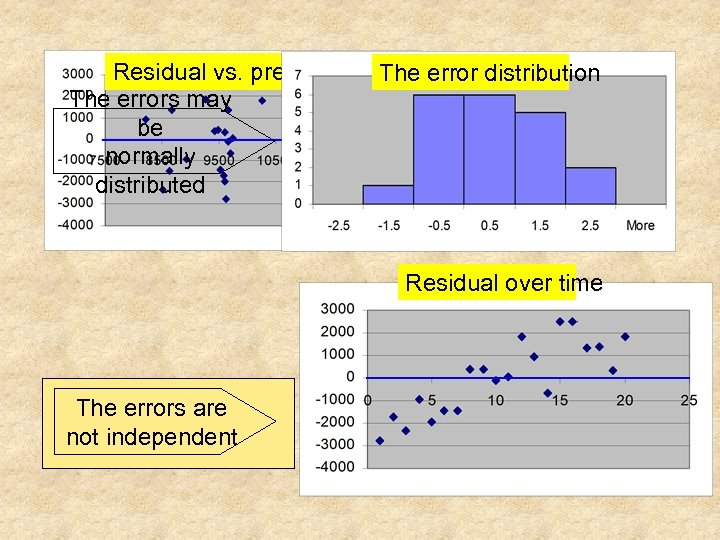Residual vs. predicted y The error distribution The errors may variance be is constant normally distributed Residual over time The errors are not independent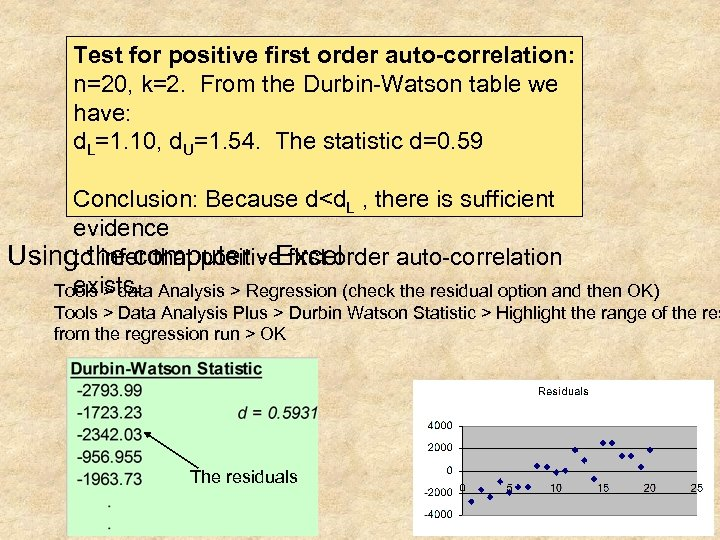Test for positive first order auto-correlation: n=20, k=2. From the Durbin-Watson table we have: d. L=1. 10, d. U=1. 54. The statistic d=0. 59 Conclusion: Because d data Analysis > Regression (check the residual option and then OK) Tools > Data Analysis Plus > Durbin Watson Statistic > Highlight the range of the res from the regression run > OK The residuals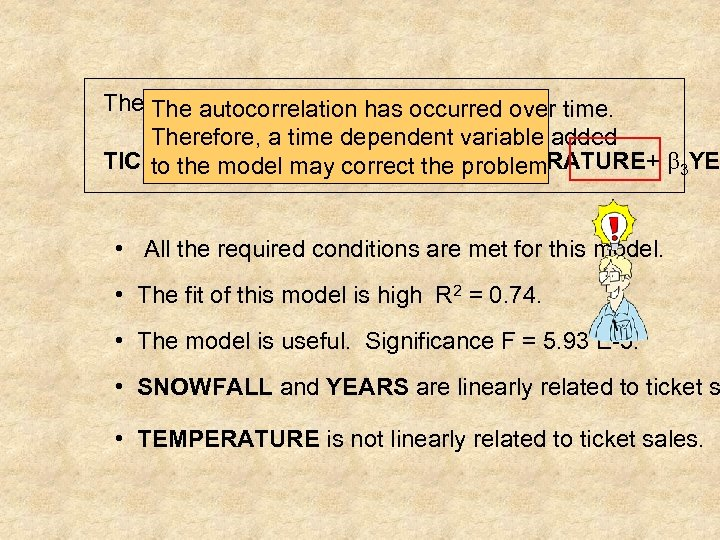The autocorrelation has occurred over time. modified regression model Therefore, a time dependent variable added TICKETS=b 0+ b 1 SNOWFALL+ b 2 problem to the model may correct the TEMPERATURE+ b 3 YE • All the required conditions are met for this model. • The fit of this model is high R 2 = 0. 74. • The model is useful. Significance F = 5. 93 E-5. • SNOWFALL and YEARS are linearly related to ticket s • TEMPERATURE is not linearly related to ticket sales.